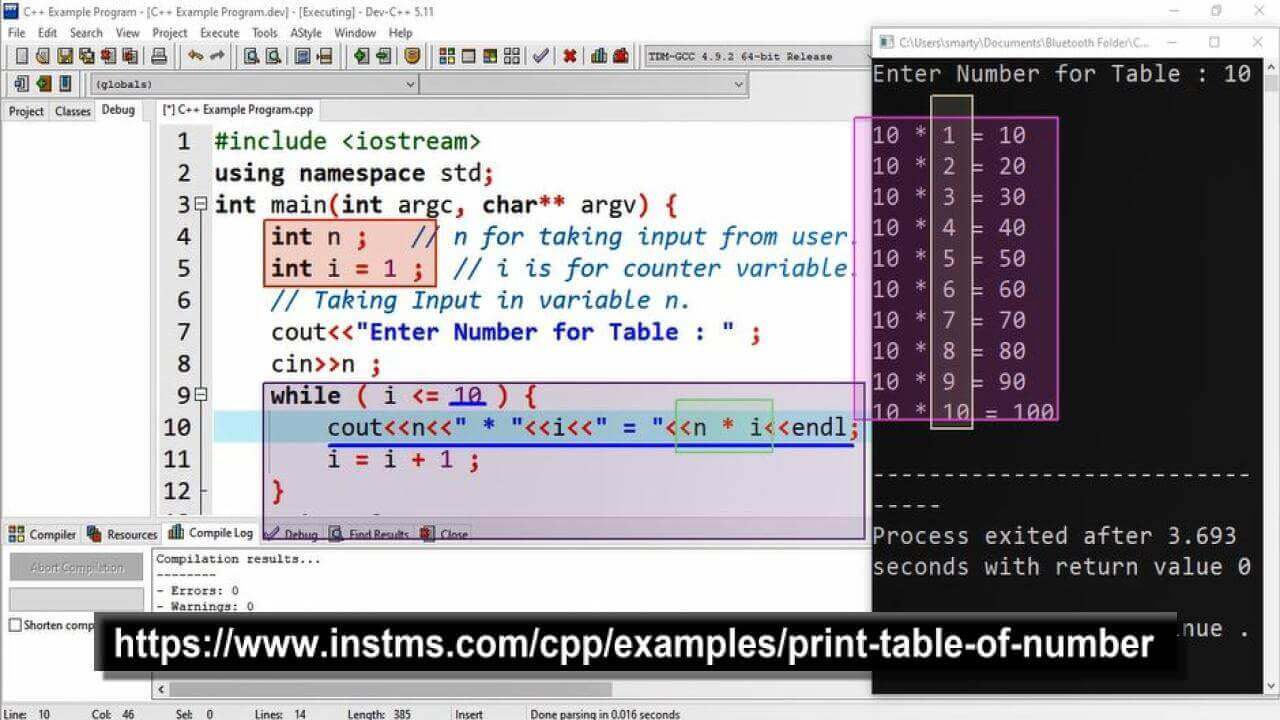# Print Table Of Number using CPP

## Source Code

#include <iostream>

using namespace std;

int main(int argc, char** argv) {
int n ;   // n for taking input from user.
int i = 1 ;  // i is for counter variable.

// Taking Input in variable n.
cout<<"Enter Number for Table : " ;
cin>>n ;

while ( i <= 10 ) {
cout<<n<<" * "<<i<<" = "<<n * i<<endl ;
i = i + 1 ;
}
return 0;
}

## Output## Working:

In this program example only two variables are declared to implement the entire logic. One variable is used to take input from the user and the second variable is used as counter variable. While loop is used for iterations. The entire logic of table program is hidden in the cout statement, where we print value of input variable, value of counter variable and their multiplication.

While loop print cout statement 10 times because table is the result of 10 muliplied iteration by the input variable N.